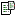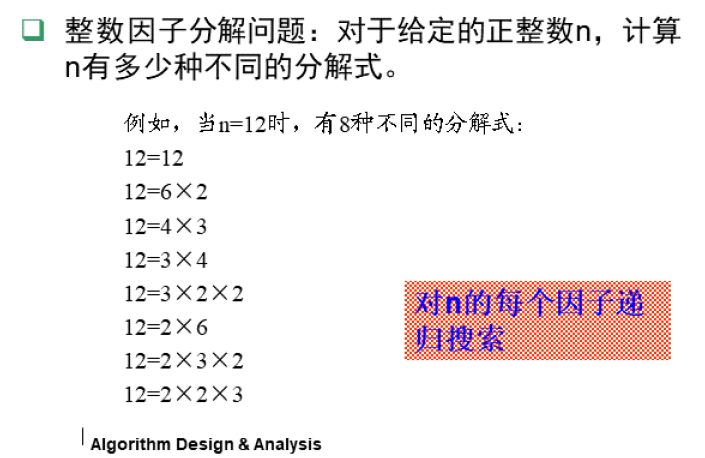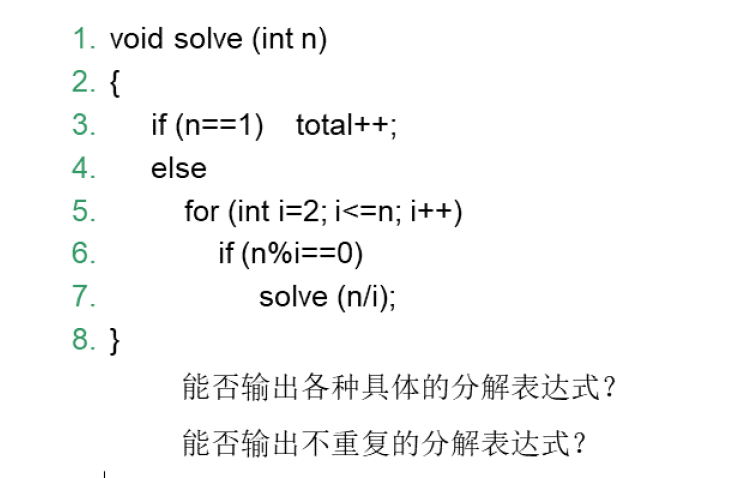• 整数因子分解问题 算法设计思路： n=x1*x2*x3*…*xm，分治思想设计（分解过程）： n=x1*(x2*x3*…*xm); n=x1*x2*(x3*…*xm); … n=x1*x2*x3*…*xm; 分治过程： void factor(int n){ int i; if(n==1)total++; else ...整数因子分解
• 对于给定的正整数n，编程计算n共有多少种不同的分解式。由文件input.txt给出输入数据。第一行有1 个正整数n (1≤n≤2000000000)。将计算出的不同的分解式总数输出到文件output.txt。
• 包含两个代码，一个是分治法求格雷码，一个是分治法求整数因子分解问题 注释详细 实现很完美 用的python 直接pycharm打开就能用
• 利用C++实现整数因子分解问题，通过input.txt文件输入数据，最终的结果输出到output.txt文件中，对该过程有较好的理解
• 程序代码：/*E-mail: sunkai [at] msn [dot] com问题描述：大于1的正整数n可以分解为n=x1 * x2 * ... * xn例如n=12时12=1212=6*212=4*312=3*412=3*2*212=2*612=2*3*212=2*2*31<=n<=2000000000*//*分析：DP之...程序代码：/*

E-mail: sunkai [at] msn [dot] com

问题描述：

大于1的正整数n可以分解为n=x1 * x2 * ... * xn

例如n=12时

12=12

12=6*2

12=4*3

12=3*4

12=3*2*2

12=2*6

12=2*3*2

12=2*2*3

1<=n<=2000000000

*/

/*

分析：

DP之记忆化搜索

状态若用d则内存不足

因此采用结构体记录状态

经过简单数学推理，对于一个数N,它的因子数不超过N^(1/2)+1

*/

#include

#include

struct DP

{

int num;

int sum;

} d={0};

int max=0;

void qsort(int low,int high,struct DP key[])

{

int i=low,j=high;

struct DP tag=key[i];

if(i

{

do

{

while(tag.num

if(i

{

key[i]=key[j];

i++;

while(tag.num>=key[i].num && i

if(i

{

key[j]=key[i];

j--;

}

}

}while(i

key[i]=tag;

qsort(low,j-1,key);

qsort(i+1,high,key);

}

}

int dfs(int left)

{

int i,p;

int l,r,m;

int count=0;

l=0; r=max;

while(l<=r)

{

m=(l+r)>>1;

if(d[m].num

}

p=l; if(d[p].sum) return d[p].sum;

for(i=1;i<=d[i].num;i++)

{

if(left%d[i].num==0) count+=dfs(left/d[i].num);

}

d[p].sum=count;

return count;

}

int main(void)

{

int i,j,tmp;

int n;

scanf("%d",&n); tmp=sqrt(n);

for(i=1;i<=tmp;i++)

{

if(n%i==0)

{

d[max].num=i; max++;

d[max].num=n/i; max++;

}

} max--;

qsort(0,max,d);

d.sum=1;

printf("%d\n",dfs(n));

return 0;

}

[[it] 本帖最后由 卧龙孔明 于 2008-3-21 20:26 编辑 [/it]]

展开全文• 9718 整数因子分解 时间限制:1000MS 内存限制:1000K 提交次数:0 通过次数:0 题型: 编程题 语言: 无限制 Description 大于1的正整数 n 都可以分解为 n = x1 * x2 * ... * xm 例如：当n=12时，共有8种不同的分解...
• Description 大于1的正整数 n 都可以分解为 n = x1 * x2 * ......递归实现整数因子分解的计数。 假设对正整数n的因子分解计数为solve(n)。 1）当n=1时，计数加1。 2）当n>1时，对每个因子i，计算solve(n/i)。
• 整数因子分解问题及其扩展问题的解答

整数因子分解问题，对于给定的正整数n，计算n有多少种不同的分解式上面已经给出了基本的方法，问题分析：这个问题其实很简单，将一个数n从2到它本身依次求余，如果发现n求余后为0，证明这个被求余的数i是这个整数的因子，那么我们对n/i再进行递归，直到n/i变为1停止递归。

扩展问题一：能否输出各种具体的分解表达式？
思路：可以设置一个栈，如果是因子，则将这个因子压入栈中，递归到因子为1时分解完毕，将整个栈中元素输出。一次递归结束后将栈顶的元素弹出。代码如下：

void calculate(int n, Stack *Top){

if( n == 1 ){
count++ ;
Print(Top);
}

for(int i=2;i<=n;i++)
{
if( n%i == 0 ){
Push(Top, i) ;
calculate( n/i, Top ) ;
Pop(Top) ;
}
}

}

这里是自定义的栈，栈的实现代码如下：

//栈的结点类型
typedef struct Node
{
int data;
struct Node *next;
}Stack;

//初始化一个栈
Stack *InitStack()
{
Stack *Top;
Top = (Stack *)malloc(sizeof(Stack));
Top->next = NULL;
}
//判断栈空
int isEmpty(Stack *Top)
{
if(Top->next==NULL)
return 0;
else
return 1;
}
//入栈
int Push(Stack *Top,int x)
{
Stack *p;
p = (Stack *)malloc(sizeof(Stack));
p->data = x;
p->next = Top->next;
Top->next = p;
return TRUE;
}
//出栈
int Pop(Stack *Top)
{
if(Top->next==NULL){
printf("ERROR\n");
return FLASE;
}
else{
Stack *p;
p = Top->next;
Top->next = p->next;
free(p);
return TRUE;
}
}
//打印栈中元素
void Print(Stack *Top)
{
Stack *p = Top->next;
while (p!=NULL){
printf("%d ",p->data);
p = p->next;
}
printf("\n") ;
}

此处没使用STL中的stack，一个主要的原因，就是我想要打印但是不清空栈，这个问题没有解决，STL中无法在不清空栈的情况下直接遍历栈。

扩展问题二：能否输出不重复的分解表达式？

第一种思路：
经过多次试验发现，如果递归结束时，模拟栈中的元素是无序的，则本次分解一定重复。以12为例，有3种情况为：2×2×3、2×3×2、3×2×2，后两种之所以重复，是因为它们都是无序的，因此，在上问题一的基础上，只须在输出之前判断一下模拟栈中的元素是否有序便可，若序时，才进行输出。代码如下:

void calculate(int n, Stack *Top){

if( n == 1 ){
count++ ;
if(isOrder(Top))
{
Print(Top);
}
}

for(int i=2;i<=n;i++)
{
if( n%i == 0 ){
Push(Top, i) ;
calculate( n/i, Top ) ;
Pop(Top) ;
}
}

}

其中判断是否有序的函数如下：

bool isOrder(Stack *Top)
{
Stack *p = Top->next;

while(p->next!=NULL)
{
Stack *q = p->next;
if(q->data > p->data)
{
return false;
}
p=p->next;
}
return true;
}

第二种思路：第一种思路的改进
既然为了保持模拟栈中元素的顺序，那每次i入栈之前先同栈顶元素进行比较，如果i大于栈顶元素，则不入栈，这种方法更简洁。代码如下：

void calculate3(int n, Stack *Top){

if( n == 1 ){
count++;
Print(Top);
}
else{
for(int i=2;i<=n;i++)
{
if( n%i == 0 ){
if(Top->next!=NULL && i<Top->next->data)
{
continue;
}
Push(Top, i) ;
calculate3( n/i, Top ) ;
Pop(Top) ;
}
}
}

}

进一步进行优化：
其实函数内层循环中i没有必要循环到n，只须要循环到sqrt(n)便可，当然，需要再补上缺失的一种情况，即当i为n乘1的情况，代码如下：

void calculate(int n, Stack *Top){
...
else{
for(int i=2;i<=sqrt(n);i++)
{
...
}
//以下三行代码，处理1乘n的情况
Push(Top, n);
calculate2(1, Top);
Pop(Top);

}
}

基于c解答的完整代码如下：

#include <stdio.h>
#include <stdlib.h>
#include <math.h>
#define TRUE  1
#define FLASE 0

int count = 0 ;
//栈的结点类型
typedef struct Node
{
int data;
struct Node *next;
}Stack;

//初始化一个栈
Stack *InitStack()
{
Stack *Top;
Top = (Stack *)malloc(sizeof(Stack));
Top->next = NULL;
}
//判断栈空
int isEmpty(Stack *Top)
{
if(Top->next==NULL)
return 0;
else
return 1;
}
//入栈
int Push(Stack *Top,int x)
{
Stack *p;
p = (Stack *)malloc(sizeof(Stack));
p->data = x;
p->next = Top->next;
Top->next = p;
return TRUE;
}
//出栈
int Pop(Stack *Top)
{
if(Top->next==NULL){
printf("ERROR\n");
return FLASE;
}
else{
Stack *p;
p = Top->next;
Top->next = p->next;
free(p);
return TRUE;
}
}
//打印栈中元素
void Print(Stack *Top)
{
Stack *p = Top->next;
while (p!=NULL){
printf("%d ",p->data);
p = p->next;
}
printf("\n") ;
}

bool isOrder(Stack *Top)
{
Stack *p = Top->next;

while(p->next!=NULL)
{
Stack *q = p->next;
if(q->data > p->data)
{
return false;
}
p=p->next;
}
return true;
}
//扩展问题1
void calculate_all(int n, Stack *Top){

if( n == 1 ){
count++ ;
Print(Top);
}
else{
for(int i=2;i<=n;i++)
{
if( n%i == 0 ){
Push(Top, i) ;
calculate_all( n/i, Top ) ;
Pop(Top) ;
}
}
}

}
//扩展问题2，第一种思路
void calculate(int n, Stack *Top){

if( n == 1 ){

if(isOrder(Top))
{
count++ ;
Print(Top);
}

}
else{
for(int i=2;i<=n;i++)
{
if( n%i == 0 ){
Push(Top, i) ;
calculate( n/i, Top ) ;
Pop(Top) ;
}
}
}

}
//扩展问题2，第一种思路的优化，循环到sqrt(n)
void calculate2(int n, Stack *Top){

if( n == 1 ){

if(isOrder(Top))
{
count++ ;
Print(Top);
}

}
else{
for(int i=2;i<=sqrt(n);i++)
{
if( n%i == 0 ){
Push(Top, i) ;
calculate2( n/i, Top ) ;
Pop(Top) ;
}
}

Push(Top, n);
calculate2(1, Top);
Pop(Top);

}

}
//扩展问题2的第二种思路
void calculate3(int n, Stack *Top){

if( n == 1 ){
count++;
Print(Top);
}
else{
for(int i=2;i<=n;i++)
{
if( n%i == 0 ){
if(Top->next!=NULL && i<Top->next->data)
{
continue;
}
Push(Top, i) ;
calculate3( n/i, Top ) ;
Pop(Top) ;
}
}
}
}
//扩展问题2的第二种思路的优化，循环到sqrt(n)
void calculate4(int n, Stack *Top){

if( n == 1 ){
count++;
Print(Top);
}
else{
for(int i=2;i<=sqrt(n);i++)
{
if( n%i == 0 ){
if(Top->next!=NULL && i<Top->next->data)
{
continue;
}
Push(Top, i) ;
calculate4( n/i, Top ) ;
Pop(Top) ;
}
}
Push(Top, n);
calculate2(1, Top);
Pop(Top);
}

}
int main()
{
int n ;
Stack *Top = InitStack() ;

printf("请输入一个正整数:") ;
scanf("%d", &n) ;

calculate_all( n, Top) ;
printf("式子个数:%d\n", count) ;
/*
calculate( n, Top) ;
printf("式子个数:%d\n", count) ;

calculate2( n, Top) ;
printf("式子个数:%d\n", count) ;

calculate3( n, Top) ;
printf("式子个数:%d\n", count) ;

calculate4( n, Top) ;
printf("式子个数:%d\n", count) ;
*/
return 0 ;
}

参考链接：
https://blog.csdn.net/qingsong3333/article/details/7348923
https://blog.csdn.net/dms2017/article/details/89192985

展开全文算法分析与设计 整数因子分解 c
• 整数因子分解问题（递归）

千次阅读 2019-08-07 10:20:31
对于给定的正整数n，计算n有多少种不同的分解式。 例如，当n=12时，有8种不同的分解式： 12=12； 12=6×2； 12=4×3； 12=3×4； 12=3×2×2； 12=2×6； 12=2×3×2； 12=2×2×3； 根据他的规律往下递归。 n的第一...

问题描述：
对于给定的正整数n，计算n有多少种不同的分解式。

例如，当n=12时，有8种不同的分解式：
12=12；
12=6×2；
12=4×3；
12=3×4；
12=3×2×2；
12=2×6；
12=2×3×2；
12=2×2×3；

根据他的规律往下递归。
n的第一个因子可能是2~n之间的数.
例如12，它的第一个因子可能是2,3,4,6,12；
将第一个因子为2的分解个数,加上第一个因子为3的分解个数,…,直至加到第一个因子为12的分解个数.
而第一个因子为2的分解个数又是多少呢?
那就是6的分解的个数,因为12/2=6，然后继续递归求解。

#include<stdio.h>
int q(int n) {
int sum=0;
for(int i=2; i<n; i++) {
if(n%i==0) {
sum++;
sum+=q(n/i);
}
}
return sum;
}
int main() {
int n;
scanf("%d",&n);
printf("%d",q(n)+1);
}

展开全文整数因子分解 递归 C语言
• 大于1的正整数n可以分解为：n=x1×x2×…×xm。例如，当n=18时，共有8种不同的分解式。 对于给定的正整数n，计算n共有多少种不同的分解式。 /////////////////////////////////////////////////////////////////////...

大于1的正整数n可以分解为：n=x1×x2×…×xm。例如，当n=18时，共有8种不同的分解式。
对于给定的正整数n，计算n共有多少种不同的分解式。

方法：将一个数n从2到它本身依次求余，如果发现n求余i后为0，说明i是这个整数的因子，那么我们对n/i再进行递归求因子，直到n/i变为1停止递归。

//*/
#include <bits/stdc++.h>
using namespace std;

int cnt = 0 ;
stack<int> s;

void write(){
int e;
stack<int> s1;

//输出栈s，即n的因子，同时用栈s1中转
while (!s.empty()){
e=s.top();
s1.push(e);
cout<<e<<"  ";
s.pop();
}//while
cout<<endl;

//以s1为中转，s因子恢复原状
while (!s1.empty()){
e=s1.top();
s.push(e);
s1.pop();
}//while
}//write

void factorization(int n){
if(n ==1){	//分解到1，获得1种方案，输出
cnt++ ;
write();
}//if

int i = 2 ;
while (i<=n){
if(n%i==0) {		//整除，i是因子
s.push(i);		//入栈

factorization(n/i) ;	//对 n/i 进行因子分解

s.pop();		//还原，因子i归还
}//if

i++ ;
}//while
}//factorization

int main(){
int n ;

cin>>n;
cout<<endl;

factorization(n) ;

cout<<endl<<cnt;

return 0 ;
}//main

使用数组模拟栈：

//*/
#include <bits/stdc++.h> //万能头文件
using namespace std;

int cnt=0; //记录可分解方案数
int a,k=0;	//模拟栈a，栈顶指针k

void write()
{
int i;
for(i=k-1;i>=0;i--)
cout<<a[i]<<" ";
cout<<endl;
}//write

void factorization(int n)
{
if(n==1) //分解到因子为1，获得一种方案
{
cnt++; //计数增加
write(); //输出
}
int i=2; //从因子2开始测试
while(i<=n) //因子不大于数本身
{
if(n%i==0) //能整除
{
a[k++]=i; //加入因子
factorization(n/i); //对 n/i 进行因子分解
k--; //归还因子
}
i++; //因子变大，再次测试
}
}//factorization

int main(){
int n ;

cin>>n;
cout<<endl;

factorization(n); //分解因子

cout<<endl<<"可分解方案数："<<cnt;

return 0 ;
}//main

结果：展开全文c++ 算法
• Python整数因子分解

千次阅读 2019-10-04 22:24:30
整数因子分解问题 问题描述： 大于1 的正整数n 可以分解为：n=X1 X 2 …Xm。 例如，当n= 12 时，共有8 种不同的分解式： 12= 12； 12=62； 12=43； 12=34； 12=322； 12=26； 12=232； 12=223。 编程...python 算法设计与分析 整数因子分解
• B - 整数因子分解问题

千次阅读 2020-09-20 21:36:36
B - 整数因子分解问题 Description 大于1的正整数n可以分解为：n=x1*x2*…*xm。例如，当n=12 时，共有8 种不同的分解式： 12=12； 12=6*2； 12=4*3； 12=3*4； 12=3*2*2； 12=2*6； 12=2*3*2； 12=2*2*3。 对于...
• 大于1 的正整数n可以分解为：n=x1*x2*…*xm。 算法设计： 对于给定的正整数n，编程计算n共有多少种不同的分解式。 例如，当n=12 时，共有8 种不同的分解式： 12=12； 12=6*2； 12=4*3； 12=3*4； 12=3*2*2； 12=2*6...整数因子分解问题
• 整数因子分解问题（分治）

万次阅读 多人点赞 2019-04-10 20:15:26
问题描述：将一个整数分解整数因子相乘，共有多少种不同的分解式？ 问题分析：这个问题其实很简单，将一个数n从2到它本身依次求余，如果发现n求余后为0，证明这个被求余的数i是这个整数的因子，那么我们对n/i再...
• 大于1的正整数 n 都可以分解为 n = x1 * x2 * ... * xm ...递归实现整数因子分解的计数。 假设对正整数n的因子分解计数为solve(n)。 1）当n=1时，计数加1。 2）当n>1时，对每个因子i，计算solve(n/i)。
• python 递归实现整数因子分解问题 问题描述： 大于1 的正整数n 可以分解为几个因子的积，例如：12共有8 种不同的分解式： 12；62；43；34；322；26；232；223；对于给定正整数n，计算共有多少种不同的分解式。 ...python 整数因子分解
• 整数因子分解

2012-11-01 00:47:38
整数因子分解 int Fenjie(int n) { int num = 0; for (int i = 2;i;i++) { if(n%i==0) { num++; num+=Fenjie(n/i); } } return num; }整数因子分解
• h(n)为n的划分数 ...整数因子分解问题 Time Limit: 1000 ms Memory Limit: 65536 KiB Problem Description 大于1的正整数n可以分解为：n=x1x2…xm。例如，当n=12 时，共有8 种不同的分解式： 1...算法
• 整数因子分解问题 问题描述： 大于1 的正整数n可以分解为：n=x1*x2*…*xm。 算法设计： 对于给定的正整数n，编程计算n共有多少种不同的分解式。 例如，当n=12 时，共有8 种不同的分解式： 12=12； 12=6*2； 12=4*3；...
• 大于1的正整数n可以分解为：n=x1 * x2 * … * xm。 例如，当n=12 时，共有8 种不同的分解式： 12=12； 12=6 * 2； 12=4 * 3； 12=3 * 4； 12=3 * 2 * 2； 12=2 * 6； 12=2 * 3 * 2； 12=2 * 2 * 3。 对于给定的正...算法 动态规划 递归法 c++
• 　大于1 的正整数n 可以分解为：n=x1*x2*…*xm。 例如，当n=12 时，共有8 种不同的分解式： 12=12； 12=6*2； 12=4*3； 12=3*4； 12=3*2*2； 12=2*6； 12=2*3*2； 12=2*2*3 。 编程任务： 　对于给定的正...算法 分治算法 c++ csp ccf
• 算法--整数因子分解问题

千次阅读 2019-03-14 19:20:11
思路：使用一个map,int>型的数组a,将每个数的因式分解存在对应的map下,例如map,4>表示6的因式分解次数是4次，所以，map中n中的数即为结果；其他计算即为我刚开始想的差不多； #include using namespace std; ......